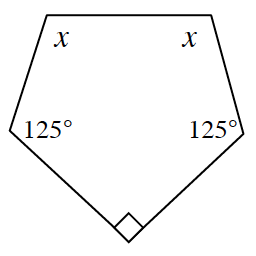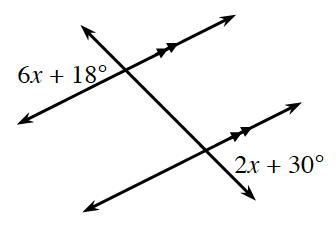### Home > CCG > Chapter Ch8 > Lesson 8.3.1 > Problem8-97

8-97.

For each diagram below, write and solve an equation to find $x$.

1.The sum of the interior angles $= 180º(n − 2)$.

$x + x + 125º + 90º + 125º =$ sum from Step 1

1.Mark the angles that are vertical to the labeled angles. What do you know about the angles that you marked?

$x = 3º$